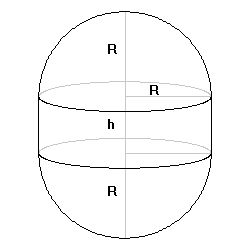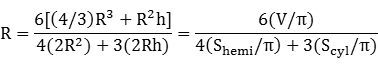Equable Capsule

EQUABLE CAPSULE

Balmoral Software

Solutions: 0Consider the capsule consisting of two hemispheres of radius R adjoining a cylinder of the same radius and height h. The total volume of the polytope is V, where
V/π = Vsphere/π + Vcylinder/π = (4/3)R3 + R2h
The surface area of each hemisphere is Shemi, where
Shemi/π = 2R2
The lateral surface area of the cylinder is Scyl, where
Scyl/π = 2Rh
The above three quantities are integers by convention. We can writeso R is rational. The equability assumption is
V = 2Shemi + Scyl,
which reduces to
3h(R - 2) = 4R(3 - R)
Incidentally, h is rational since it can be written as the quotient of rationals if R ≠ 2. Since h > 0 and R > 0, R - 2 and 3 - R must have the same sign, so
2 < R < 3

Shemi/π = 2R2 ∈ {9,10,11,12,13,14,15,16,17}

4R2 ∈ {18,20,22,24,26,28,30,32,34}

By writing the fractionwe can see that no feasible value of 4R2 produces an integer value of the numerator, so R cannot be rational, a contradiction. Therefore, there are no equable capsules with integer-valued volume and surface area sections.